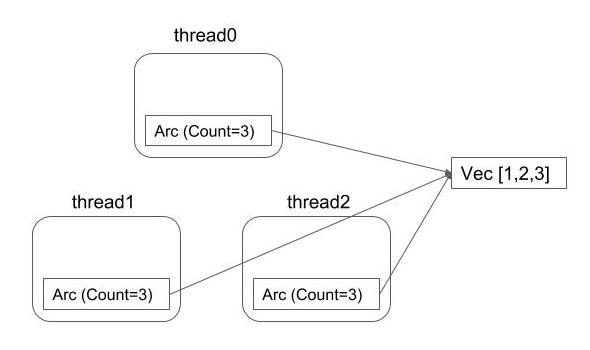# Arc<Mutex<T>> is a design pattern in Rust

Rust has no GC and you can't write some kind of code that you ever wrote in other languages. The typical case is recursive data structures like list, tree and graph.

By looking for documents or articles, you will find types like the following. You may be terrified because it seems like monad stacks in Haskell.

```enum List {
Cons(Rc<RefCell<i32>>, Rc<List>),
Nil,
}
```
```pub struct NodeRef<T>(Rc<RefCell<Node<T>>>);
```

These documents usually start from "Let's implement tree structure in Rust" , explain why these types are required and then extend it to multi-threaded variants. But in my blog however, will explain in the reverse order because I thought it would be easier to understand for readers. This article is the first step.

In my opinion, I think the nesting type `reference_counting<internal_mutability<T>>` is like a design pattern in Rust language and single-threaded and multi-threaded variants share the same intrinsics.

In this article, you will learn why the type `Arc<Mutex<T>>` is required in multi-threaded programming. Let's start from a small experiment.

### Experiment 1: You can't pass reference to thread

In this experiment, you pass a reference to a spawned thread.

```use std::thread;

fn main()
{
let v = vec![1,2,3];
println!("{:?}", v);
});
hdl.join().unwrap();
}
```

However, this code is not compilable.

```error[E0373]: closure may outlive the current function, but it borrows `v`, which is owned by the current function
--> prog.rs:6:29
|
6 |     let hdl = thread::spawn(|| {
|                             ^^ may outlive borrowed value `v`
7 |         println!("{:?}", v);
|                          - `v` is borrowed here
help: to force the closure to take ownership of `v` (and any other referenced variables), use the `move` keyword```

The error says that compiler thinks the thread is possibly running after the `v` got out of scope. For us programmers, it is not possible though, the compiler is conservative. Changing the closure to move closure as compiler's advice will succeed compile.

### Experiment 2: Spawn two threads

```use std::thread;

fn main()
{
let v = vec![1,2,3];
let hdl0 = thread::spawn(move || {
println!("{:?}", v);
});
let hdl1 = thread::spawn(move || {
println!("{:?}", v);
});

hdl0.join().unwrap();
hdl1.join().unwrap();
}
```

This is not compilable.

```error[E0382]: capture of moved value: `v`
--> prog.rs:10:26
|
6  |     let hdl0 = thread::spawn(move || {
|                              ------- value moved (into closure) here
...
10 |         println!("{:?}", v);
|                          ^ value captured here after move
|
= note: move occurs because `v` has type `std::vec::Vec<i32>`, which does not implement the `Copy` trait```

The error says that `v` is moved into the first thread and not able to capture in the second thread. As the note says, if the value is `Copy` and copy on move then the code is runnable.

### Experiment 3: Clone read-only ownership using Arc

Arc can solve this problem. Arc or Atomically Reference Counted is a smart pointer which implements thread-safe reference counting. Calling `Arc::clone` clones the ownership. So in the following code, there are totally three clones of read-only ownerships and two of the three are moved to two spawned threads. As it is reference counted, the last one to drop will drop the wrapped value.

This is like a designed pattern in Rust. The single-threaded variant `Rc` is designed in the same way.

You may call `clone` method instead to clone the ownership but calling `Arc::clone` is advised so it is obvious for code readers to find it doesn't clone the entire data but clone the ownership only.

```use std::thread;
use std::sync::Arc;

fn main()
{
let v = Arc::new(vec![1,2,3]);

let v0 = Arc::clone(&v);
let hdl0 = thread::spawn(move || {
println!("{:?}", v0);
});

let v1 = Arc::clone(&v);
let hdl1 = thread::spawn(move || {
println!("{:?}", v1);
});

hdl0.join().unwrap();
hdl1.join().unwrap();
}
```

This code compiles. And the reference counts are finally as follows:### Experiment 4: Let's mutate the value

Let's mutably borrow from `v0` and `v1`.

```use std::thread;
use std::sync::Arc;

fn main()
{
let v = Arc::new(vec![1,2,3]);

let v0 = Arc::clone(&v);
let hdl0 = thread::spawn(move || {
v0.push(4);
});

let v1 = Arc::clone(&v);
let hdl1 = thread::spawn(move || {
v1.push(5);
});

hdl0.join().unwrap();
hdl1.join().unwrap();
}
```

As you easily imagine because the `Arc` was mentioned read-only, the compiler rejects this code.

```error[E0596]: cannot borrow immutable borrowed content as mutable
--> prog.rs:10:9
|
10 |         v0.push(4);
|         ^^ cannot borrow as mutable

error[E0596]: cannot borrow immutable borrowed content as mutable
--> prog.rs:15:9
|
15 |         v1.push(5);
|         ^^ cannot borrow as mutable```

### Experiment 5: Use mutex to mutate the value behind the immutable borrow

In this the last experiment. In experiment 4, the problem was that `Arc` is only capable of returning immutable borrow. So the idea is doing some trick so it is mutable behind immutable borrow. It is `Mutex`.

```use std::thread;
use std::sync::{Arc, Mutex};

fn main()
{
let v = Arc::new(Mutex::new(vec![1,2,3]));

let v0 = Arc::clone(&v);
let hdl0 = thread::spawn(move || {
v0.lock().unwrap().push(4);
});

let v1 = Arc::clone(&v);
let hdl1 = thread::spawn(move || {
v1.lock().unwrap().push(5);
});

hdl0.join().unwrap();
hdl1.join().unwrap();

println!("{:?}", v);
}
```
`Mutex { data: [1, 2, 3, 5, 4] }`

Why `Mutex` is able to mutate the value behind the immutable borrow is that it takes exclusive lock runtime to access the value. Such like `Mutex` that doesn't use static borrow checker but do the same check in runtime is called "Internal Mutability". The single-threaded variant of `Mutex` is `RefCell`.

You can learn why `Arc<RefCell<T>>` can not be used here by reading this official document but in short, `RefCell` is not `Sync`. This will be explained in the later articles.

std::sync::Arc - Rust